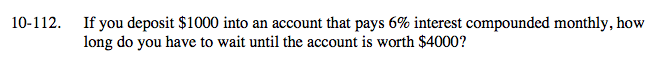### Home > A2C > Chapter Ch10 > Lesson 10.2.4 > Problem10-112

10-112.Use y = abx where:
y = amount in account (\$)
x = time (months)
a = start point = 1000
b = multiplier = .06

23.16 years